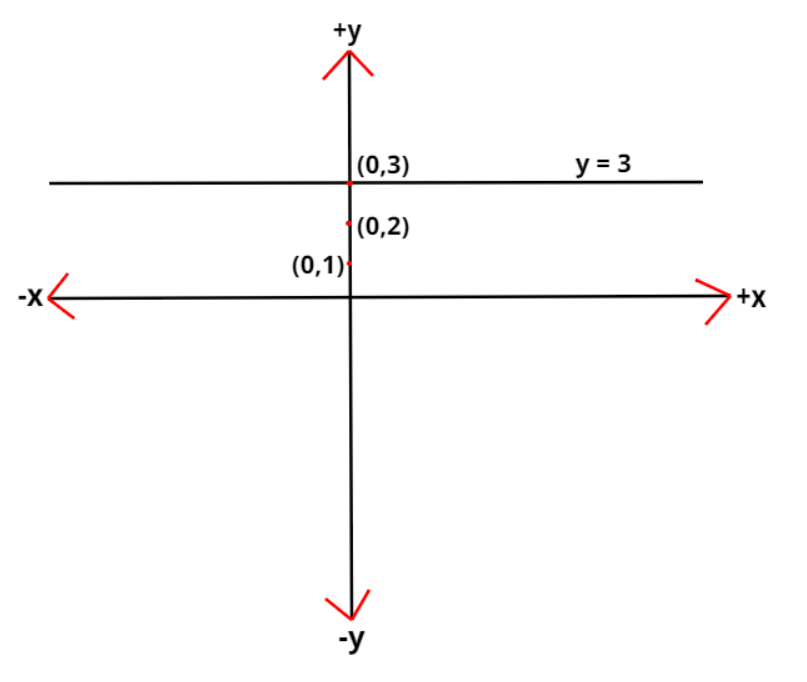Courses
Courses for Kids
Free study material
Free LIVE classes
MoreLIVE
Join Vedantu’s FREE Mastercalss

# Give geometric representation of $y = 3$ as an equation in one variable.Verified
365.1k+ views
Hint- Here, we will be comparing the given equation with the equation of any straight line.

Given equation in one variable is $y = 3$
As we know that the equation of any straight line is $y = mx + c$ where $m$ is the slope of that line and $c$ is the y-intercept of the line.
If we compare the given equation with the equation of any straight line, we can say that the given equation in one variable is the equation of a straight line with a slope of zero and y-intercept as 3 which means the given straight line is cutting the y-axis at $\left[ {0,3} \right]$.
The geometrical representation of $y = 3$ as an equation in one variable is shown in the below figure.Note- In these types of problems, we compare the given equation with any similar type of equation which is generalised for any curve in order to get a geometrical representation of the given equation.
Last updated date: 29th Sep 2023
Total views: 365.1k
Views today: 9.65k